# 实锤，无脑定投指数基金真的可以赚钱么？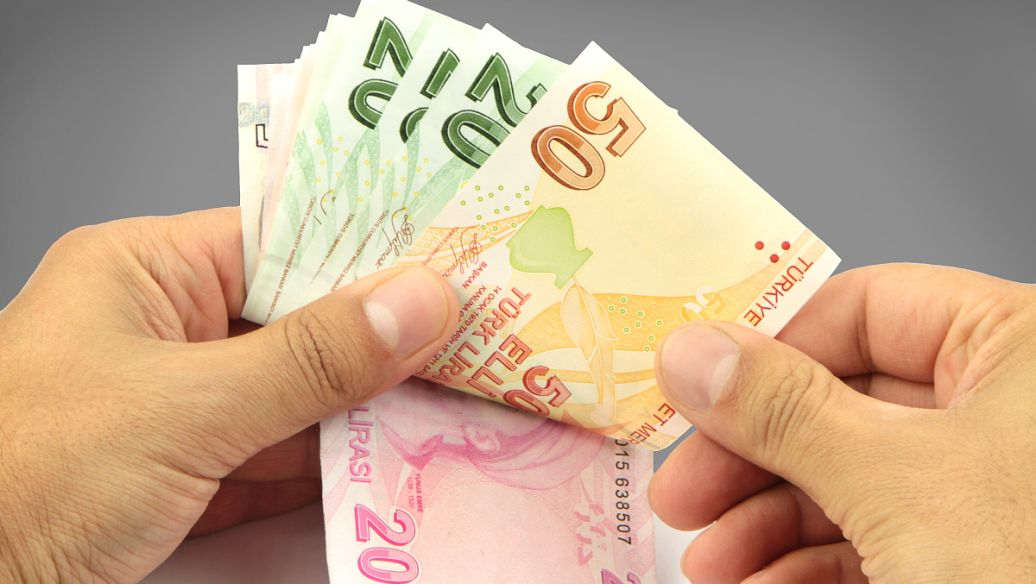## 开锤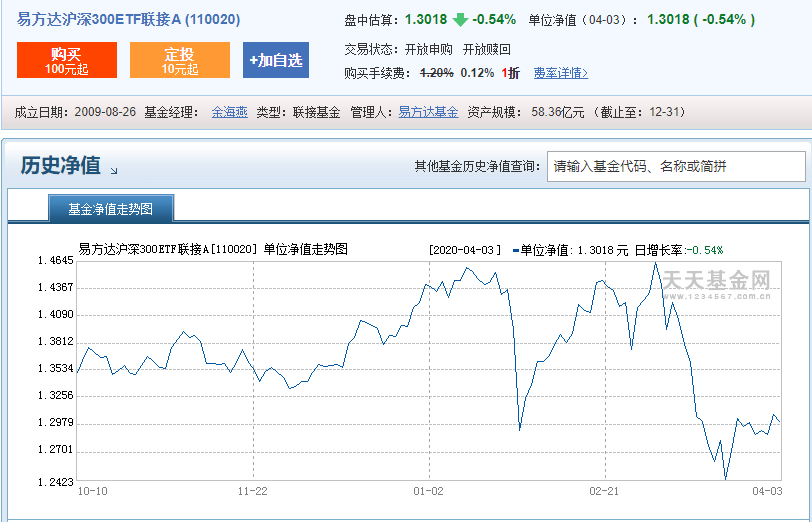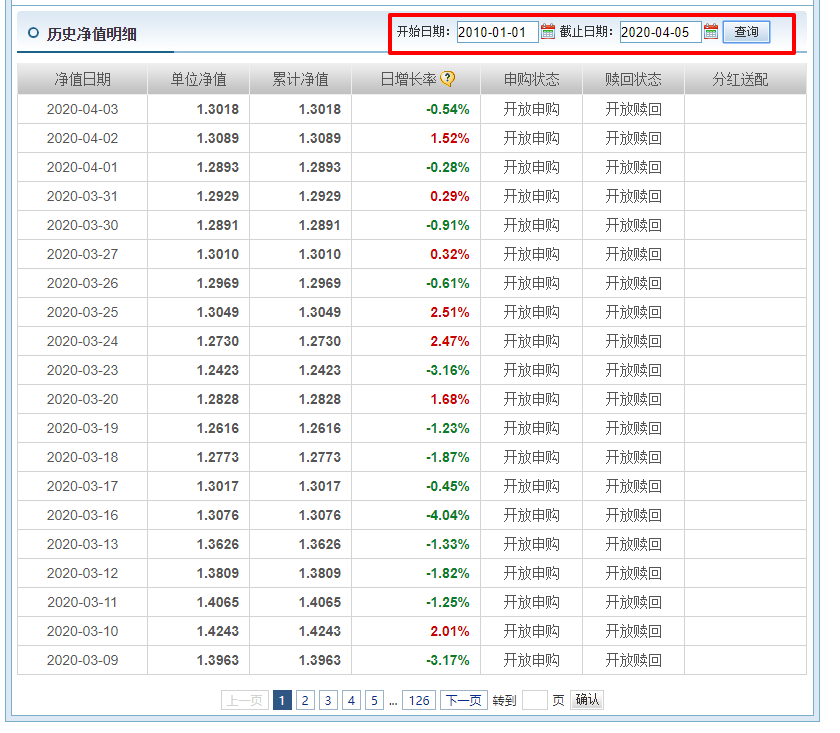http://api.fund.eastmoney.com/f10/lsjz?callback=jQuery18303890660068294629_1586089381722&fundCode=110020&pageIndex=1&pageSize=20&startDate=2010-01-01&endDate=2020-04-05&_=1586089722912


import requests

startDate = '2010-01-01'
endDate = '2020-04-05'
foundCode = '110020'
pageSize = 4000

'User-Agent': 'Mozilla/5.0 (Windows NT 10.0; Win64; x64) AppleWebKit/537.36 (KHTML, like Gecko) Chrome/80.0.3987.149 Safari/537.36',
'Referer': f'http://fundf10.eastmoney.com/jjjz_110020.html'
}

url = f'http://api.fund.eastmoney.com/f10/lsjz?&fundCode=110020&pageIndex=1&pageSize={pageSize}&startDate={startDate}&endDate={endDate}&_=1586089722912'

def write_file(content):
filename = '110020.txt'
with open(filename, 'a') as f:
f.write(content + '\n')

write_file(response.text)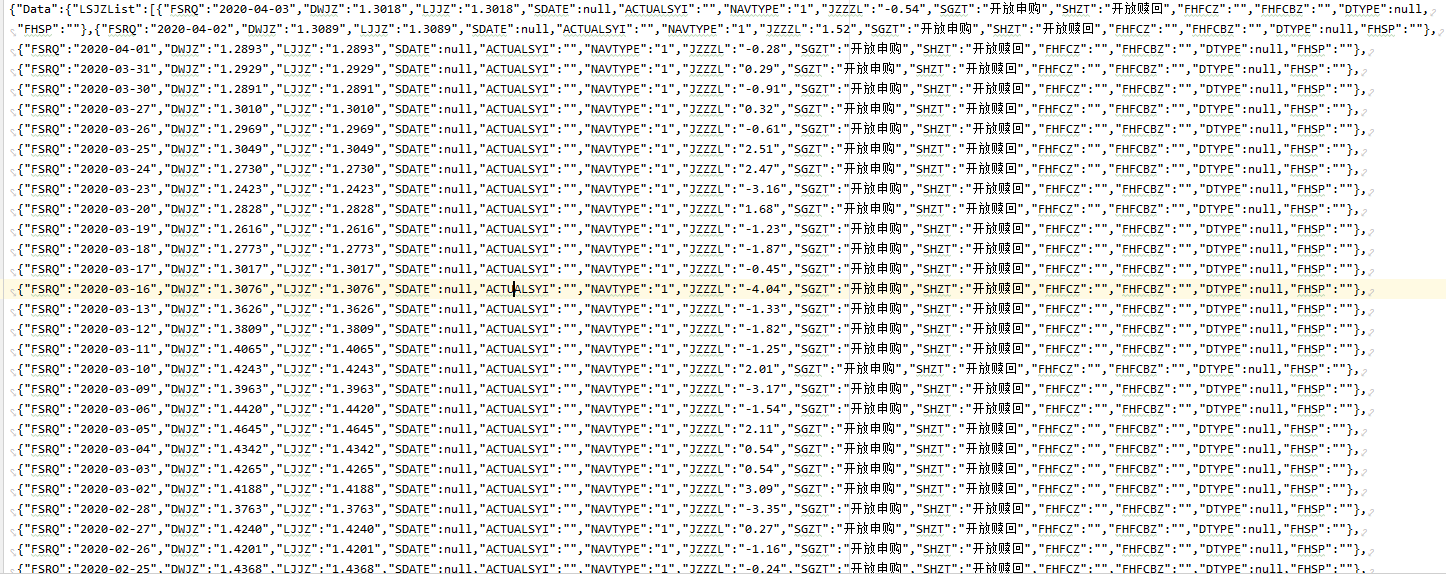## 计算收益

def get_data():
'''
获取数据
:return: dict
'''
with open('110020.txt') as f:
date_price = {}
for found in result['Data']['LSJZList'][::-1]:
date_price[found['FSRQ']] = found['DWJZ']
return date_price

date_price = get_data()

print(date_price)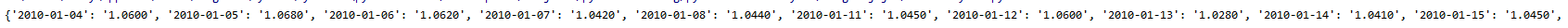def calculate_by_week(start_date, end_date):
'''
每周一定投，每次定投 500
:param start_date: 开始时间
:param end_date: 结束时间
:return:
'''
total_stock = 0
total_amount = 0
nums = 0
day = start_date + datetime.timedelta(days=-1)
while day < end_date:
day = day + datetime.timedelta(days=1)
if day.weekday() != 1:
continue
while date_price.get(day.strftime('%Y-%m-%d'), None) is None and day < end_date:
day += datetime.timedelta(days=1)
if day == end_date:
break
nums += 1
total_stock += round(500 / float(date_price[day.strftime('%Y-%m-%d')]), 2)
total_amount += 500

# 计算盈利
while date_price.get(end_date.strftime('%Y-%m-%d'), None) is None:
end_date += datetime.timedelta(days=-1)

total_profit = round(total_stock, 2) * float(date_price[end_date.strftime('%Y-%m-%d')]) - total_amount

return nums, round(total_stock, 2), total_amount, round(total_profit)


def get_first_day_of_next_month(date):
first_day = datetime.datetime(date.year, date.month, 1)
days_num = calendar.monthrange(first_day.year, first_day.month)  # 获取一个月有多少天
return first_day + datetime.timedelta(days=days_num)

def calculate_by_month(start_date, end_date):
'''
按月定投，每月 1 号买入，如果 1 号不是交易日，则顺延至下一交易日
:param start_date:
:param end_date:
:return:
'''
total_stock = 0
total_amount = 0
nums = 0
first_day = datetime.datetime(start_date.year, start_date.month, 1)
day = first_day + datetime.timedelta(days=-1)  # 将日期设置为 start_date 上个月最后一天
while day < end_date:
day = get_first_day_of_next_month(day)
while date_price.get(day.strftime('%Y-%m-%d'), None) is None and day < end_date:
day = day + datetime.timedelta(days=1)
if day == end_date:
break
nums += 1
if day.strftime('%Y-%m-%d') in date_price:
total_stock += round(2000 / float(date_price[day.strftime('%Y-%m-%d')]), 2)
total_amount += 2000

# 计算盈利
while date_price.get(end_date.strftime('%Y-%m-%d'), None) is None:
end_date += datetime.timedelta(days=-1)

total_profit = round(total_stock, 2) * float(date_price[end_date.strftime('%Y-%m-%d')]) - total_amount

return nums, round(total_stock, 2), total_amount, round(total_profit)


## 数据分析

line = (
Line()
'',
y_axis=list(date_price.values())
)
.set_global_opts(
title_opts=opts.TitleOpts(title='110020 基金走势图'),
)
)
line.render()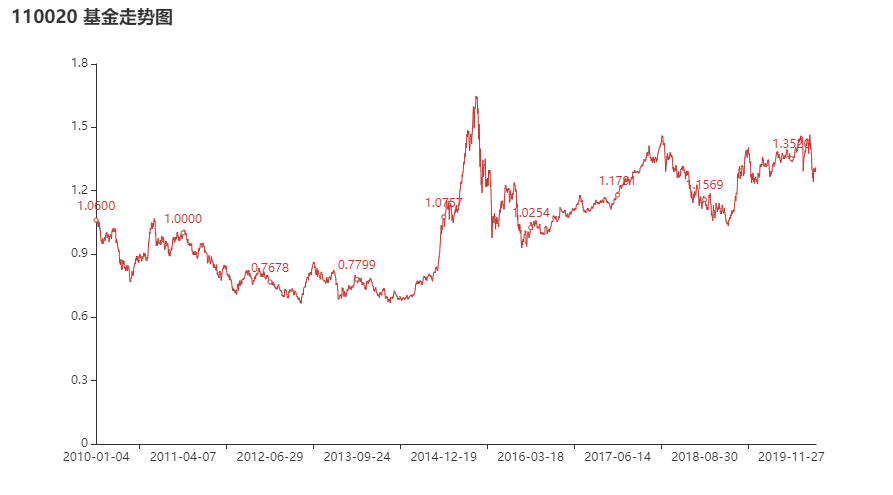# 按周定投
print(calculate_by_week(datetime.datetime.strptime(startDate,'%Y-%m-%d').date(), datetime.datetime.strptime(endDate,'%Y-%m-%d').date()))
# 按月定投
print(calculate_by_month(datetime.datetime.strptime(startDate,'%Y-%m-%d'), datetime.datetime.strptime(endDate,'%Y-%m-%d')))


(533, 273836.56, 266500, 89980)
(125, 254918.67, 250000, 81853)


10 年 33% ，好像收益比余额宝差不多了，不过这里还有一个概念，就是我们这笔钱是逐年投入的，而不是一次性投入，实际上收益应该是个 2 倍左右的关系。这个和分期还款的利息算法差不多，不多说。

# 2015年开始，按周定投
print(calculate_by_week(datetime.datetime.strptime('2015-01-01','%Y-%m-%d').date(), datetime.datetime.strptime(endDate,'%Y-%m-%d').date()))
# 2015年开始，按月定投
print(calculate_by_month(datetime.datetime.strptime('2015-01-01','%Y-%m-%d'), datetime.datetime.strptime(endDate,'%Y-%m-%d')))


(273, 113522.13, 136500, 11283)
(65, 106851.87, 130000, 9100)


## 示例代码03-3015204-223万+
08-1887
03-1648
12-041万+
01-23401
11-16163
07-29163
02-19282
01-0449
07-0894
09-1173
01-23509
11-12142
07-0961
07-28
01-213946
03-06
01-182万+
05-12点击重新获取扫码支付余额充值